# SAT II Math II : Solving Equations

## Example Questions

2 Next →

### Example Question #11 : Solving Equations

Solve the equation: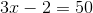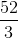Explanation: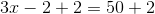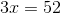Divide by three on both sides.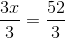The answer is:### Example Question #14 : Single Variable Algebra

Solve: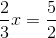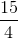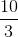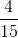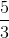Explanation:

To isolate the x-variable, multiply both sides by the coefficient of the x-variable.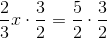The answer is:### Example Question #12 : Solving Equations

Give the solution set of the following rational equation: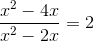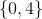No solution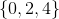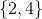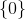No solution

Explanation:

Multiply both sides of the equation by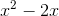to eliminate the fraction: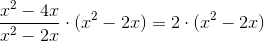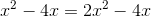Subtract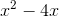from both sides: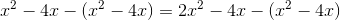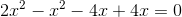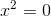The only possible solution is, However, if this is substituted in the original equation, the expression at left is undefined, as seen here: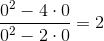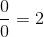An expression with a denominator of 0 has an undefined value, so this statement is false. The equation has no solution.

### Example Question #11 : Solving Equations

Give the set of all real solutions of the following equation: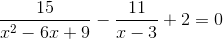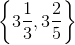None of these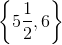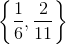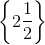Explanation: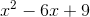can be seen to fit the perfect square trinomial pattern: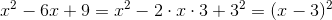The equation can therefore be rewritten as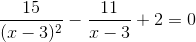Multiply both sides of the equation by the least common denominator of the expressions, which is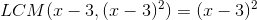: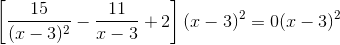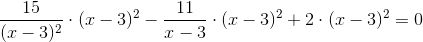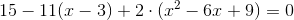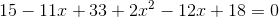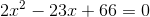This can be solved using the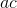method. We are looking for two integers whose sum is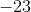and whose product is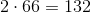. Through some trial and error, the integers are found to beand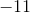, so the above equation can be rewritten, and solved using grouping, as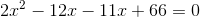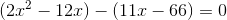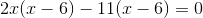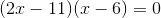By the Zero Product Principle, one of these factors is equal to zero:

Either: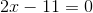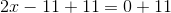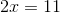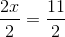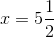Or: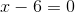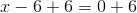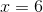Both solutions can be confirmed by substitution; the solution set is.

### Example Question #21 : Single Variable Algebra

Solve: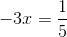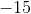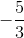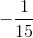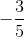Explanation:

To solve for x, multiply by negative one-third on both sides.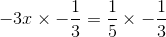The answer is:### Example Question #11 : Solving Equations

Solve the equation: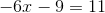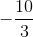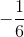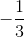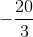Explanation: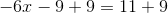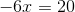Divide by negative six on both sides.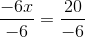The answer is:2 Next →

### All SAT II Math II Resources